Скачать презентацию Physics of Technology PHYS 1800 Lecture 23 First

18ee4cc708c14613ddb7879eeaf5d9d8.ppt

• Количество слайдов: 46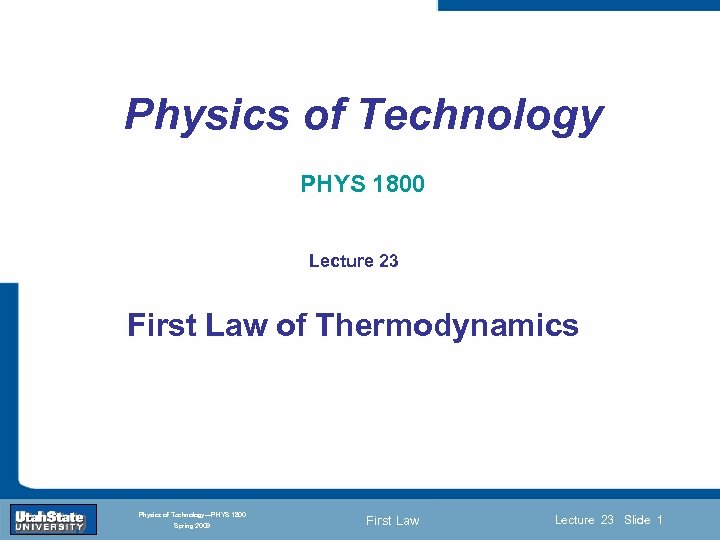Physics of Technology PHYS 1800 Lecture 23 First Law of Thermodynamics Introduction Section 0 Lecture 1 Slide 1 INTRODUCTION TO Modern Physics PHYX 2710 Fall 2004 Physics of Technology—PHYS 1800 Spring 2009 First Law Lecture 23 Slide 1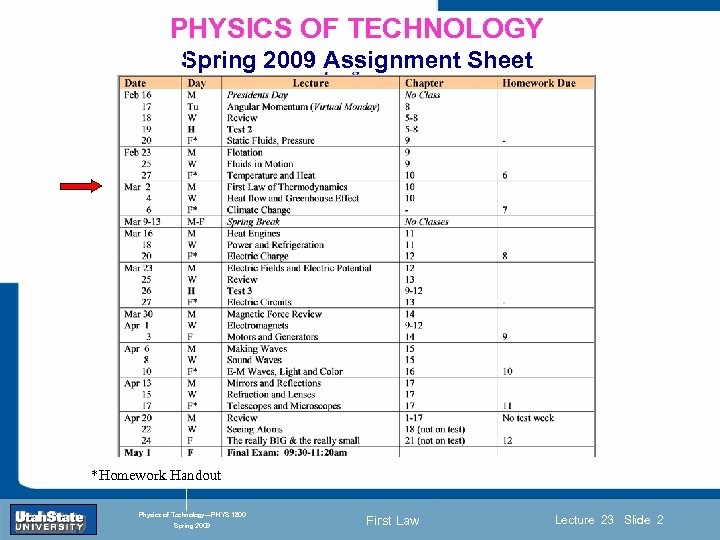PHYSICS OF TECHNOLOGY Spring 2009 Assignment Sheet Introduction Section 0 Lecture 1 Slide 2 INTRODUCTION TO Modern Physics PHYX 2710 Fall 2004 *Homework Handout Physics of Technology—PHYS 1800 Spring 2009 First Law Lecture 23 Slide 2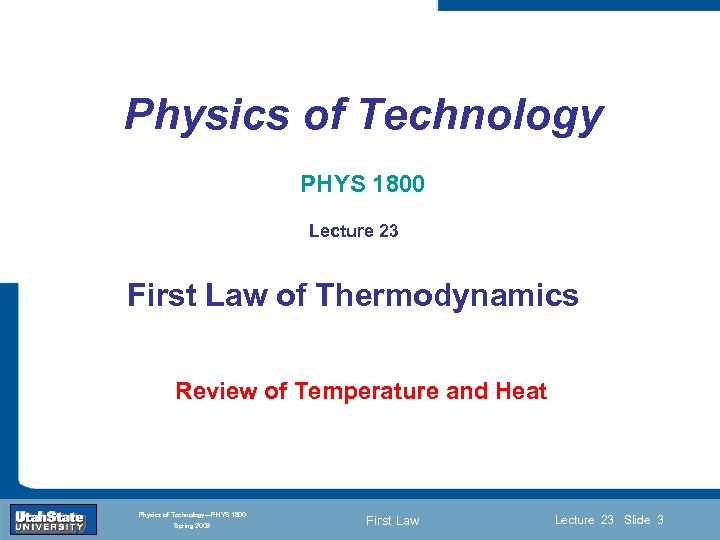Physics of Technology PHYS 1800 Lecture 23 First Law of Thermodynamics Section 0 Review Lecture 1 Slide 3 of Temperature and Heat Introduction INTRODUCTION TO Modern Physics PHYX 2710 Fall 2004 Physics of Technology—PHYS 1800 Spring 2009 First Law Lecture 23 Slide 3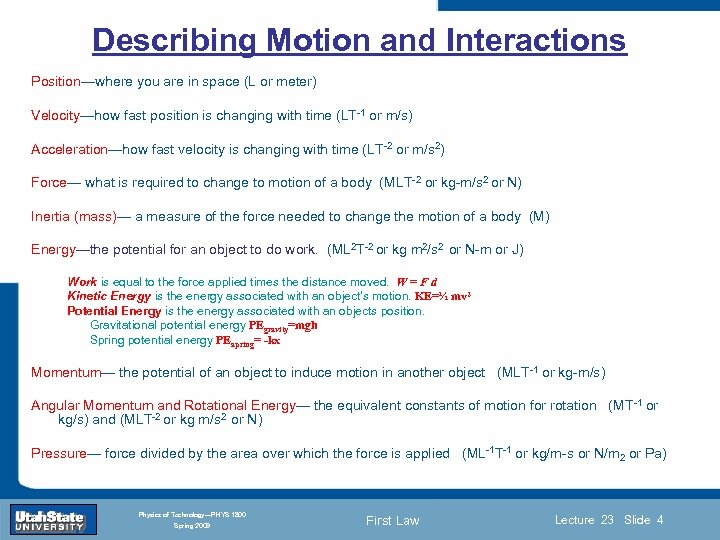Describing Motion and Interactions Position—where you are in space (L or meter) Velocity—how fast position is changing with time (LT-1 or m/s) Acceleration—how fast velocity is changing with time (LT-2 or m/s 2) Force— what is required to change to motion of a body (MLT-2 or kg-m/s 2 or N) Inertia (mass)— a measure of the force needed to change the motion of a body (M) Energy—the potential for an object to do work. (ML 2 T-2 or kg m 2/s 2 or N-m or J) Work is equal to the force applied times the distance moved. W = F d Kinetic Energy is the energy associated with an object’s motion. KE=½ mv 2 Potential Energy is the energy associated with an objects position. Gravitational potential energy PEgravity=mgh Spring potential energy PEapring= -kx Momentum— the potential of an object to induce motion in another object (MLT-1 or kg-m/s) Introduction Section 0 Lecture 1 Slide 4 Angular Momentum and Rotational Energy— the equivalent constants of motion for rotation (MT-1 or kg/s) and (MLT-2 or kg m/s 2 or N) INTRODUCTION TO Modern Physics PHYX 2710 Pressure— force divided by 2004 area over which the force is applied (ML-1 T-1 or kg/m-s or N/m 2 or Pa) the Fall Physics of Technology—PHYS 1800 Spring 2009 First Law Lecture 23 Slide 4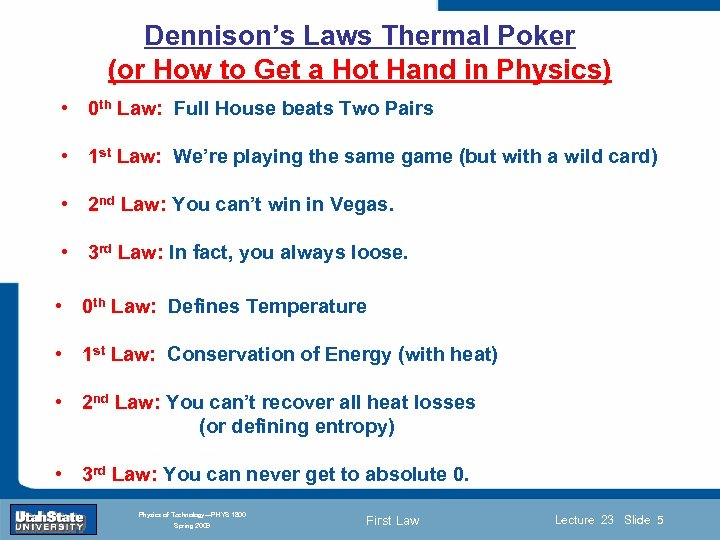Dennison’s Laws Thermal Poker (or How to Get a Hot Hand in Physics) • 0 th Law: Full House beats Two Pairs • 1 st Law: We’re playing the same game (but with a wild card) • 2 nd Law: You can’t win in Vegas. • 3 rd Law: In fact, you always loose. • 0 th Law: Defines Temperature • 1 st Law: Conservation of Energy (with heat) • 2 nd Introduction Section 0 Lecture 1 Slide 5 Law: You can’t recover all heat losses (or defining entropy) INTRODUCTION TO Modern Physics PHYX 2710 • 3 rd Fall 2004 Law: You can never get to absolute 0. Physics of Technology—PHYS 1800 Spring 2009 First Law Lecture 23 Slide 5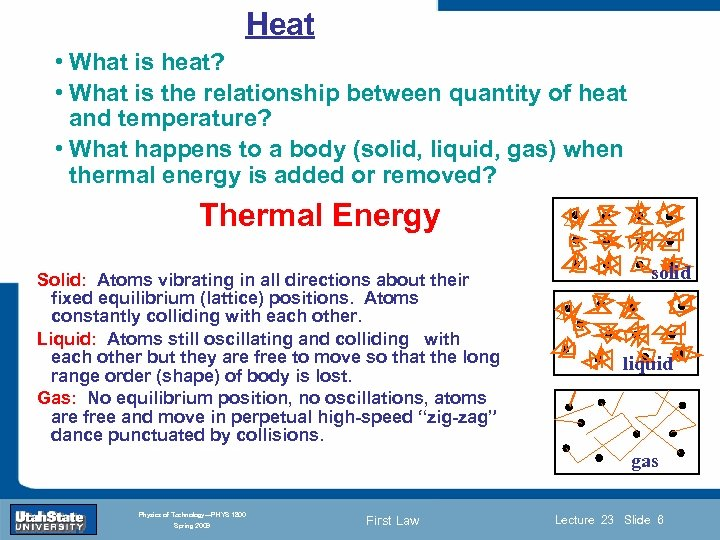Heat • What is heat? • What is the relationship between quantity of heat and temperature? • What happens to a body (solid, liquid, gas) when thermal energy is added or removed? Thermal Energy Solid: Atoms vibrating in all directions about their fixed equilibrium (lattice) positions. Atoms constantly colliding with each other. Liquid: Atoms still oscillating and colliding with each other but they are free to move so that the long range order (shape) of body is lost. Introduction Section 0 Lecture 1 Slide 6 Gas: No equilibrium position, no oscillations, atoms are free and move in perpetual high-speed “zig-zag” dance punctuated by collisions. INTRODUCTION TO Modern Physics PHYX 2710 Spring 2009 liquid gas Fall 2004 Physics of Technology—PHYS 1800 solid First Law Lecture 23 Slide 6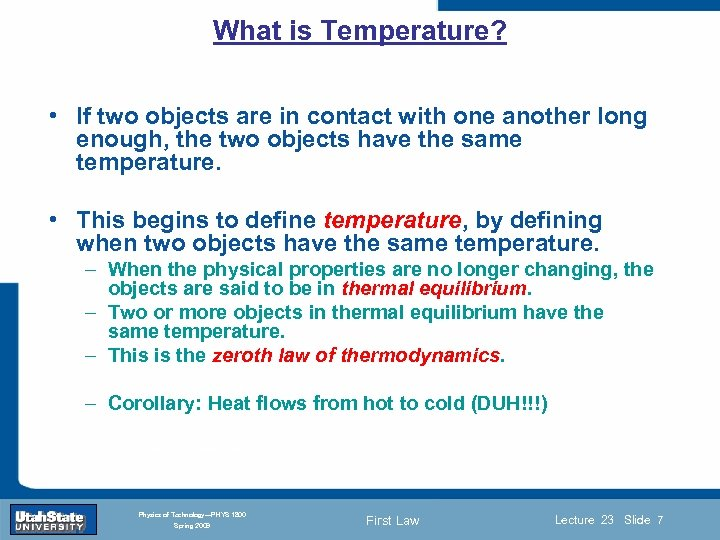What is Temperature? • If two objects are in contact with one another long enough, the two objects have the same temperature. • This begins to define temperature, by defining when two objects have the same temperature. – When the physical properties are no longer changing, the objects are said to be in thermal equilibrium. – Two or more objects in thermal equilibrium have the same temperature. – This is the zeroth law of thermodynamics. Introduction Section 0 Lecture 1 Slide 7 – Corollary: Heat flows from hot to cold (DUH!!!) INTRODUCTION TO Modern Physics PHYX 2710 Fall 2004 Physics of Technology—PHYS 1800 Spring 2009 First Law Lecture 23 Slide 7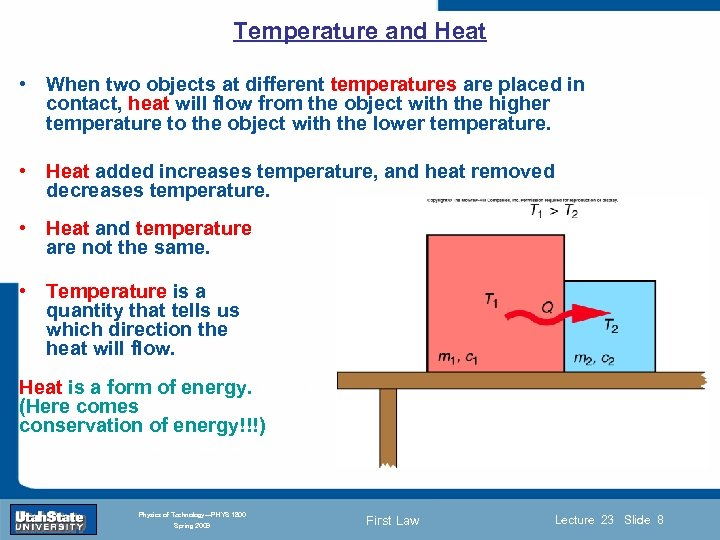Temperature and Heat • When two objects at different temperatures are placed in contact, heat will flow from the object with the higher temperature to the object with the lower temperature. • Heat added increases temperature, and heat removed decreases temperature. • Heat and temperature are not the same. • Temperature is a quantity that tells us which direction the heat will flow. Introduction Section 0 Heat is a form of energy. Lecture (Here comes conservation of energy!!!) 1 Slide 8 INTRODUCTION TO Modern Physics PHYX 2710 Fall 2004 Physics of Technology—PHYS 1800 Spring 2009 First Law Lecture 23 Slide 8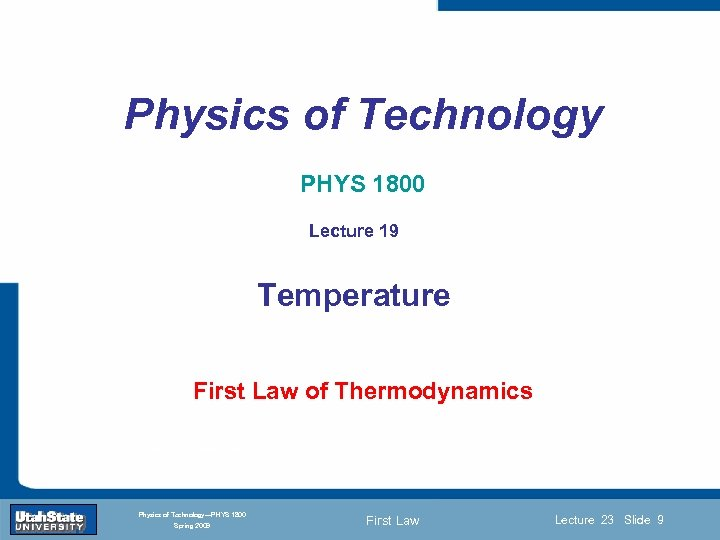Physics of Technology PHYS 1800 Lecture 19 Temperature Introduction Section 0 Lecture 1 First Law of Slide 9 Thermodynamics INTRODUCTION TO Modern Physics PHYX 2710 Fall 2004 Physics of Technology—PHYS 1800 Spring 2009 First Law Lecture 23 Slide 9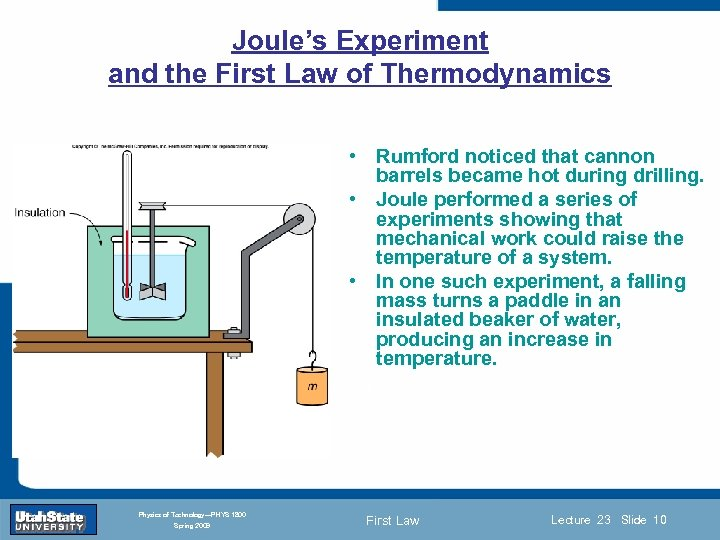Joule’s Experiment and the First Law of Thermodynamics • Rumford noticed that cannon barrels became hot during drilling. • Joule performed a series of experiments showing that mechanical work could raise the temperature of a system. • In one such experiment, a falling mass turns a paddle in an insulated beaker of water, producing an increase in temperature. Introduction Section 0 Lecture 1 Slide 10 INTRODUCTION TO Modern Physics PHYX 2710 Fall 2004 Physics of Technology—PHYS 1800 Spring 2009 First Law Lecture 23 Slide 10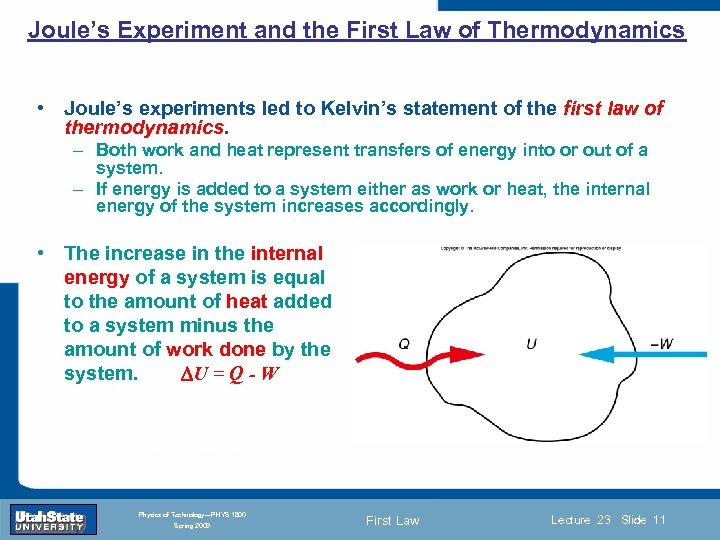Joule’s Experiment and the First Law of Thermodynamics • Joule’s experiments led to Kelvin’s statement of the first law of thermodynamics. – Both work and heat represent transfers of energy into or out of a system. – If energy is added to a system either as work or heat, the internal energy of the system increases accordingly. • The increase in the internal energy of a system is equal to the amount of heat added to a system minus the amount of work done by the system. U = Q - W Introduction Section 0 Lecture 1 Slide 11 INTRODUCTION TO Modern Physics PHYX 2710 Fall 2004 Physics of Technology—PHYS 1800 Spring 2009 First Law Lecture 23 Slide 11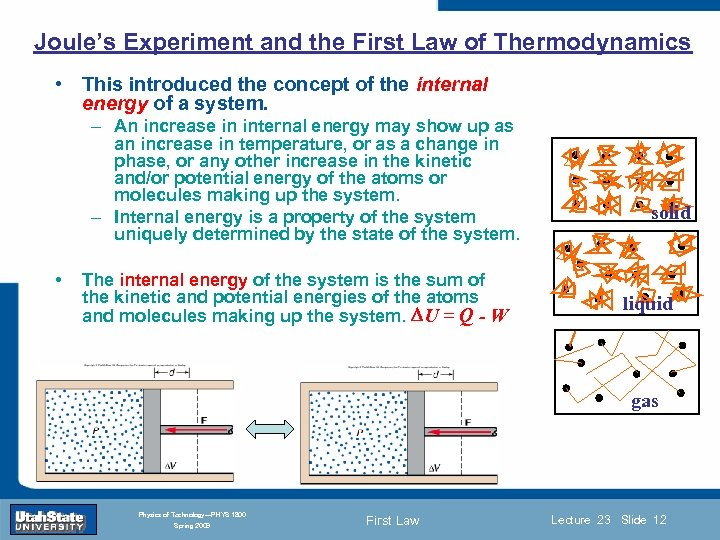Joule’s Experiment and the First Law of Thermodynamics • This introduced the concept of the internal energy of a system. – An increase in internal energy may show up as an increase in temperature, or as a change in phase, or any other increase in the kinetic and/or potential energy of the atoms or molecules making up the system. – Internal energy is a property of the system uniquely determined by the state of the system. • The internal energy of the system is the sum of the kinetic and potential energies of the atoms and molecules making up the system. U = Q - W Introduction Section 0 Lecture 1 Slide 12 solid liquid gas INTRODUCTION TO Modern Physics PHYX 2710 Fall 2004 Physics of Technology—PHYS 1800 Spring 2009 First Law Lecture 23 Slide 12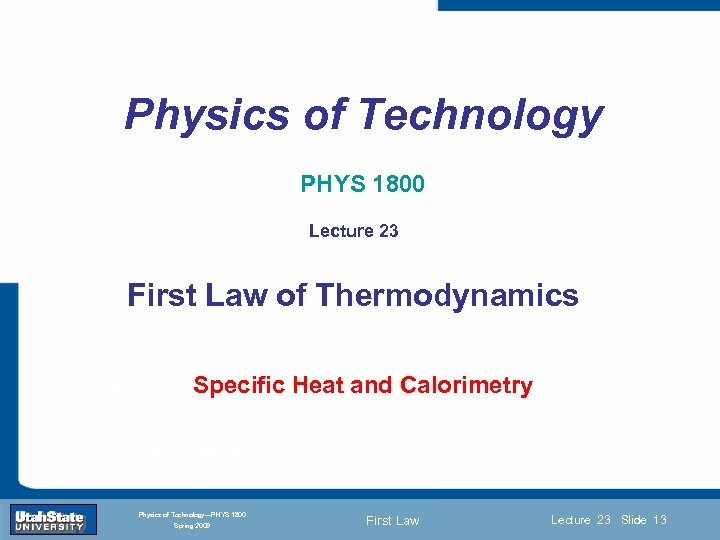Physics of Technology PHYS 1800 Lecture 23 First Law of Thermodynamics Introduction Lecture 1 Slide 13 Specific Heat and Calorimetry Section 0 INTRODUCTION TO Modern Physics PHYX 2710 Fall 2004 Physics of Technology—PHYS 1800 Spring 2009 First Law Lecture 23 Slide 13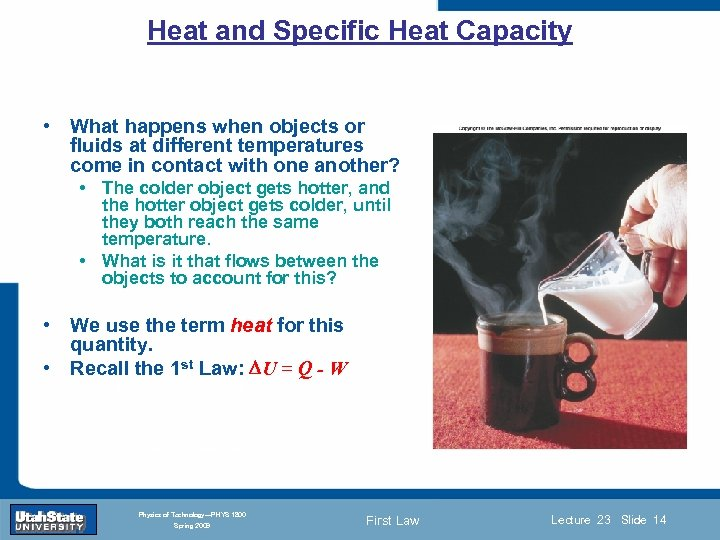Heat and Specific Heat Capacity • What happens when objects or fluids at different temperatures come in contact with one another? • The colder object gets hotter, and the hotter object gets colder, until they both reach the same temperature. • What is it that flows between the objects to account for this? • We use the term heat for this quantity. • Recall the 1 st Law: U = Q - W Introduction Section 0 Lecture 1 Slide 14 INTRODUCTION TO Modern Physics PHYX 2710 Fall 2004 Physics of Technology—PHYS 1800 Spring 2009 First Law Lecture 23 Slide 14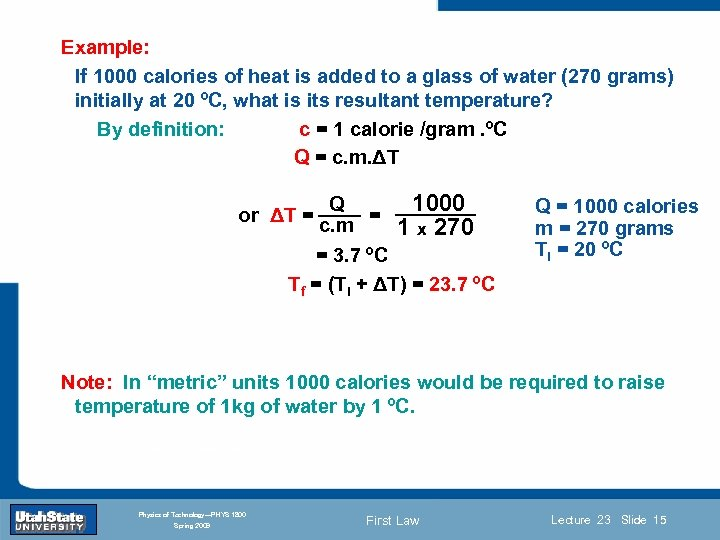Example: If 1000 calories of heat is added to a glass of water (270 grams) initially at 20 ºC, what is its resultant temperature? By definition: c = 1 calorie /gram. ºC Q = c. m. ΔT or ΔT = Q = c. m 1000 1 x 270 = 3. 7 ºC Tf = (Ti + ΔT) = 23. 7 ºC Q = 1000 calories m = 270 grams Ti = 20 ºC Note: Introduction Sectionunits 1000 Slide 15 In “metric” 0 Lecture 1 calories would be required to raise temperature of 1 kg of water by 1 ºC. INTRODUCTION TO Modern Physics PHYX 2710 Fall 2004 Physics of Technology—PHYS 1800 Spring 2009 First Law Lecture 23 Slide 15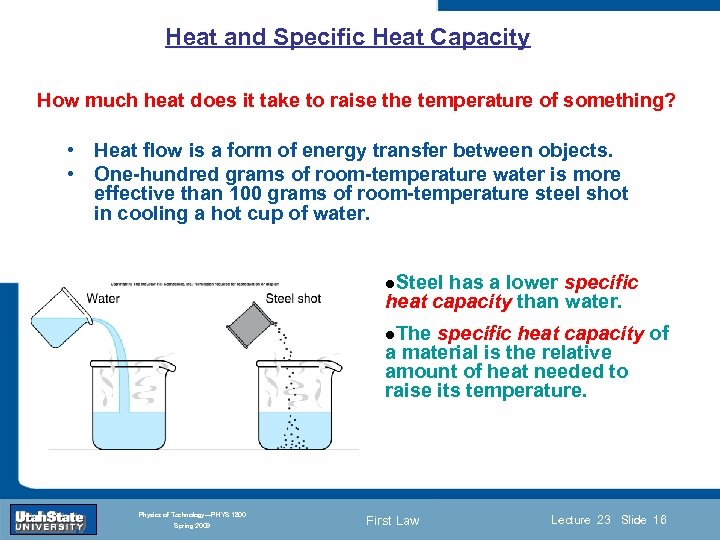Heat and Specific Heat Capacity How much heat does it take to raise the temperature of something? • Heat flow is a form of energy transfer between objects. • One-hundred grams of room-temperature water is more effective than 100 grams of room-temperature steel shot in cooling a hot cup of water. l. Steel has a lower specific heat capacity than water. l. The Introduction Section 0 Lecture 1 Slide 16 specific heat capacity of a material is the relative amount of heat needed to raise its temperature. INTRODUCTION TO Modern Physics PHYX 2710 Fall 2004 Physics of Technology—PHYS 1800 Spring 2009 First Law Lecture 23 Slide 16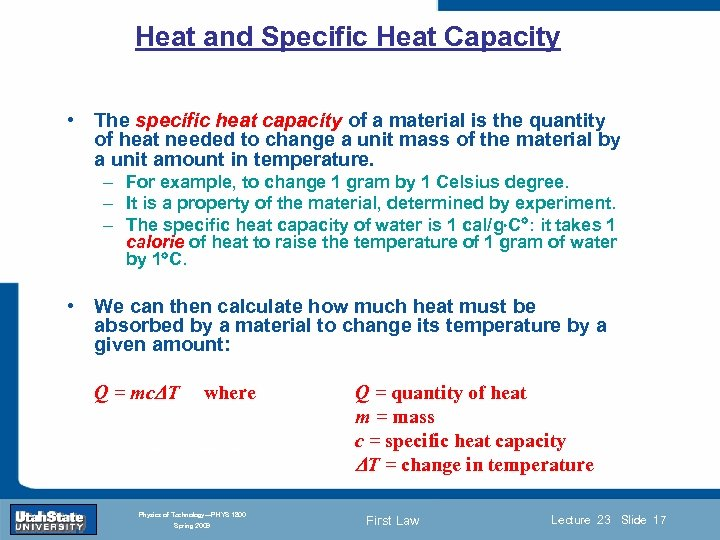Heat and Specific Heat Capacity • The specific heat capacity of a material is the quantity of heat needed to change a unit mass of the material by a unit amount in temperature. – For example, to change 1 gram by 1 Celsius degree. – It is a property of the material, determined by experiment. – The specific heat capacity of water is 1 cal/g C : it takes 1 calorie of heat to raise the temperature of 1 gram of water by 1 C. • We can then calculate how much heat must be absorbed by a material to change its temperature by a given amount: Introduction Q = mc T Section 0 where Lecture INTRODUCTION TO Modern Physics PHYX 2710 Fall 2004 Physics of Technology—PHYS 1800 Spring 2009 1 Slide 17 Q = quantity of heat m = mass c = specific heat capacity T = change in temperature First Law Lecture 23 Slide 17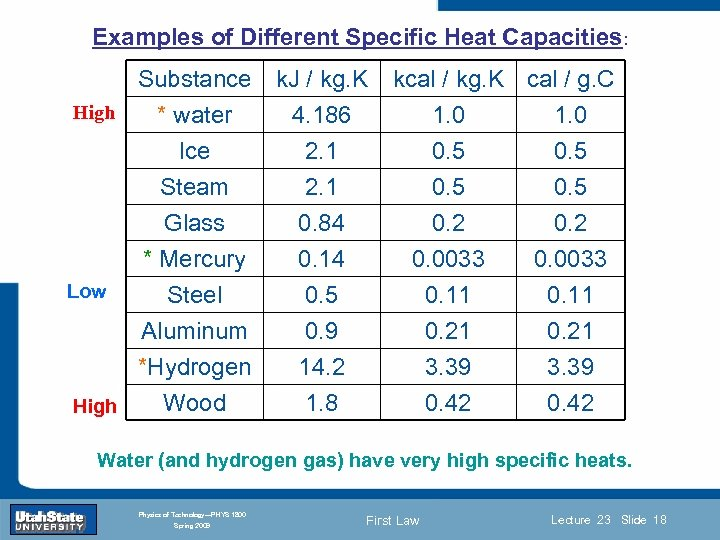Examples of Different Specific Heat Capacities: Substance High * water Ice Steam k. J / kg. K 4. 186 2. 1 Glass * Mercury Low Steel Aluminum *Hydrogen Introduction Section 0 Wood High 0. 84 0. 14 0. 5 0. 9 14. 2 Lecture 1 Slide 18 1. 8 kcal / kg. K cal / g. C 1. 0 0. 5 0. 2 0. 0033 0. 11 0. 21 3. 39 0. 42 INTRODUCTION TO Modern Physics PHYX 2710 Water (and hydrogen gas) have very high specific heats. Fall 2004 Physics of Technology—PHYS 1800 Spring 2009 First Law Lecture 23 Slide 18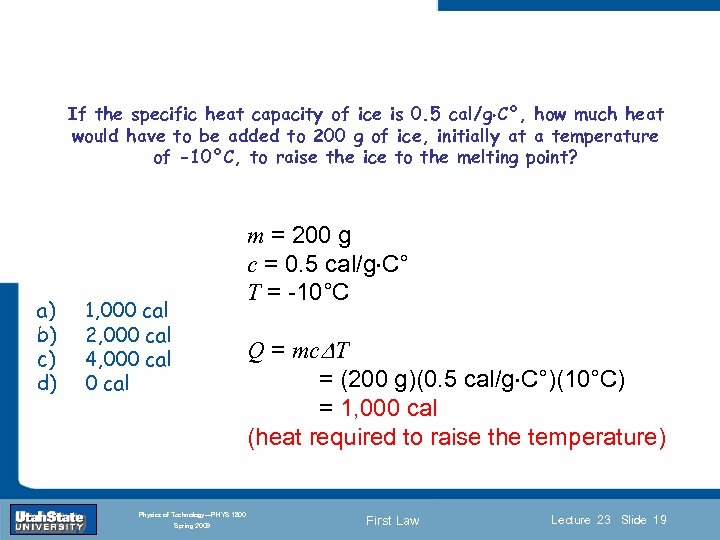If the specific heat capacity of ice is 0. 5 cal/g C°, how much heat would have to be added to 200 g of ice, initially at a temperature of -10°C, to raise the ice to the melting point? a) b) c) d) 1, 000 cal 2, 000 cal 4, 000 cal Introduction m = 200 g c = 0. 5 cal/g C° T = -10°C Q = mc T Section 0 Lecture 1 = (200 g)(0. 5 cal/g C°)(10°C) Slide 19 = 1, 000 cal (heat required to raise the temperature) INTRODUCTION TO Modern Physics PHYX 2710 Fall 2004 Physics of Technology—PHYS 1800 Spring 2009 First Law Lecture 23 Slide 19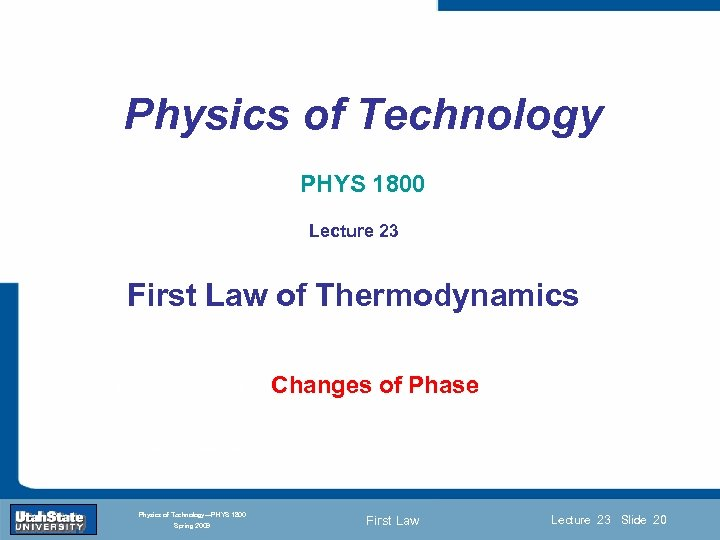Physics of Technology PHYS 1800 Lecture 23 First Law of Thermodynamics Introduction Section 0 Slide 20 Changes of Phase Lecture 1 INTRODUCTION TO Modern Physics PHYX 2710 Fall 2004 Physics of Technology—PHYS 1800 Spring 2009 First Law Lecture 23 Slide 20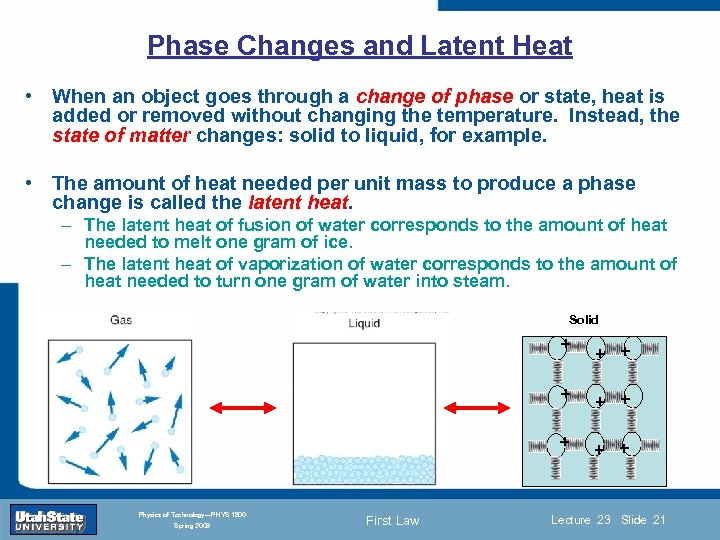Phase Changes and Latent Heat • When an object goes through a change of phase or state, heat is added or removed without changing the temperature. Instead, the state of matter changes: solid to liquid, for example. • The amount of heat needed per unit mass to produce a phase change is called the latent heat. – The latent heat of fusion of water corresponds to the amount of heat needed to melt one gram of ice. – The latent heat of vaporization of water corresponds to the amount of heat needed to turn one gram of water into steam. + INTRODUCTION TO Modern Physics PHYX 2710 Fall 2004 Physics of Technology—PHYS 1800 Spring 2009 First Law + Slide 21 + + + Lecture 1 + Section 0 + Introduction + + Solid Lecture 23 Slide 21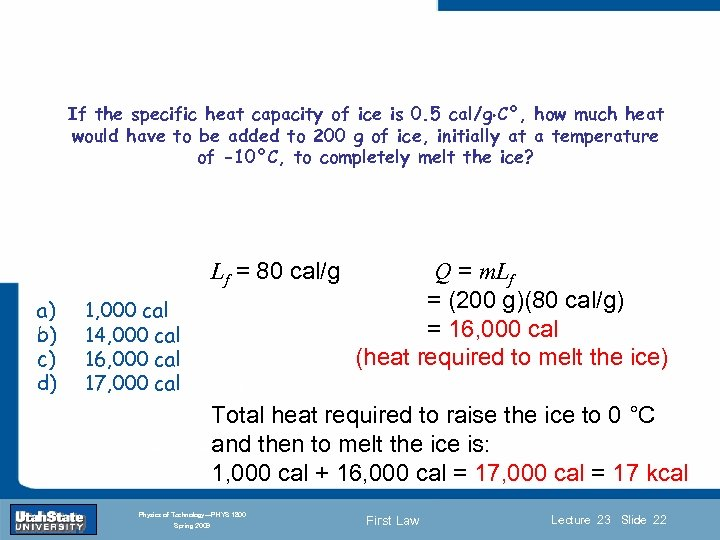If the specific heat capacity of ice is 0. 5 cal/g C°, how much heat would have to be added to 200 g of ice, initially at a temperature of -10°C, to completely melt the ice? Lf = 80 cal/g a) b) c) d) 1, 000 cal 14, 000 cal 16, 000 cal 17, 000 cal Introduction Section 0 Lecture 1 Q = m. Lf = (200 g)(80 cal/g) = 16, 000 cal (heat required to melt the ice) Slide 22 Total heat required to raise the ice to 0 °C and then to melt the ice is: 1, 000 cal + 16, 000 cal = 17 kcal INTRODUCTION TO Modern Physics PHYX 2710 Fall 2004 Physics of Technology—PHYS 1800 Spring 2009 First Law Lecture 23 Slide 22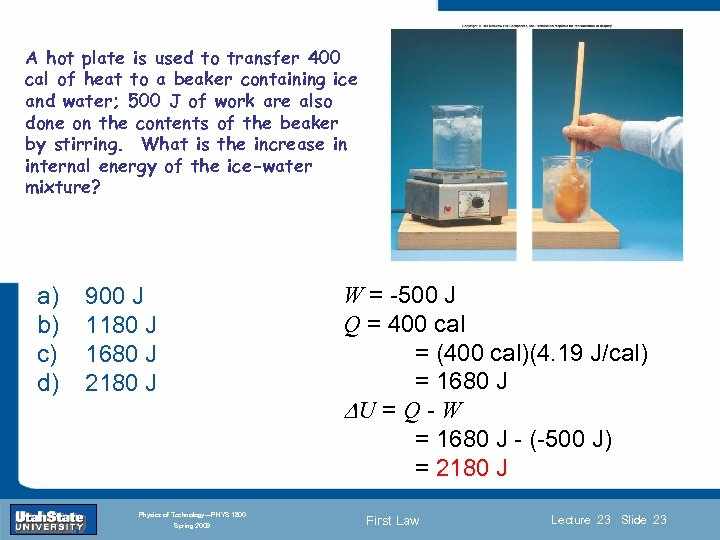A hot plate is used to transfer 400 cal of heat to a beaker containing ice and water; 500 J of work are also done on the contents of the beaker by stirring. What is the increase in internal energy of the ice-water mixture? a) b) c) d) 900 J 1180 J 1680 J 2180 J Introduction Section 0 Lecture 1 INTRODUCTION TO Modern Physics PHYX 2710 Fall 2004 Physics of Technology—PHYS 1800 Spring 2009 W = -500 J Q = 400 cal = (400 cal)(4. 19 J/cal) = 1680 J Slide 23 U = Q - W = 1680 J - (-500 J) = 2180 J First Law Lecture 23 Slide 23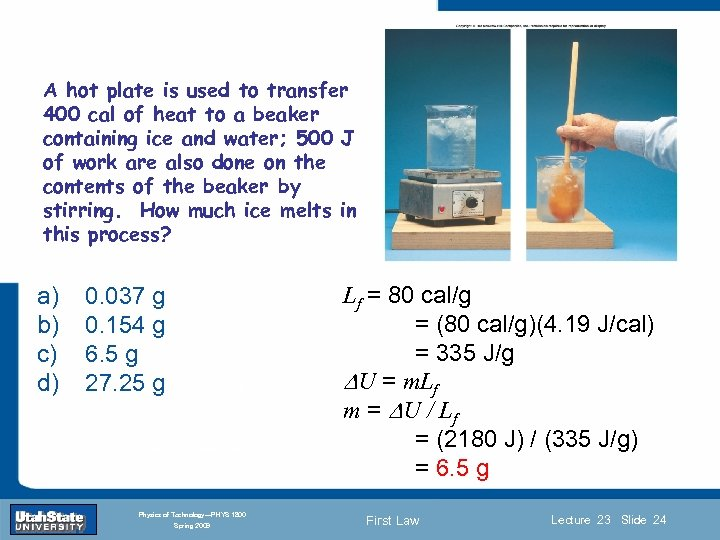A hot plate is used to transfer 400 cal of heat to a beaker containing ice and water; 500 J of work are also done on the contents of the beaker by stirring. How much ice melts in this process? a) b) c) d) 0. 037 g 0. 154 g 6. 5 g 27. 25 g Introduction Section 0 Lecture 1 INTRODUCTION TO Modern Physics PHYX 2710 Fall 2004 Physics of Technology—PHYS 1800 Spring 2009 Lf = 80 cal/g = (80 cal/g)(4. 19 J/cal) = 335 J/g 24 Slide U = m. Lf m = U / Lf = (2180 J) / (335 J/g) = 6. 5 g First Law Lecture 23 Slide 24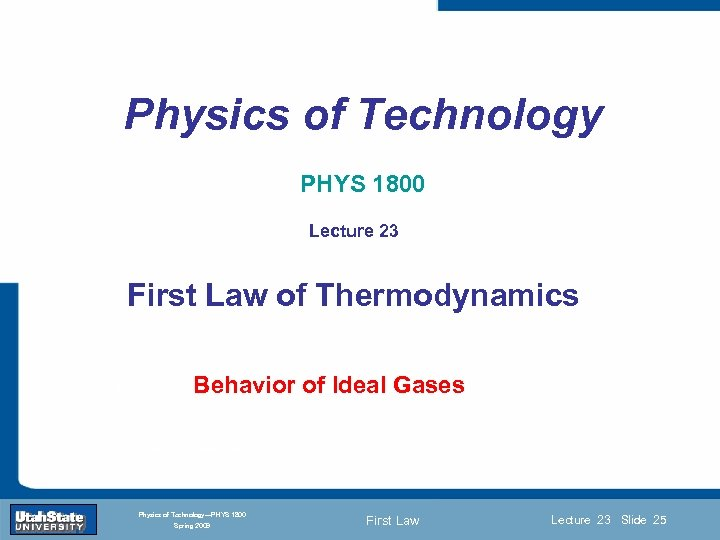Physics of Technology PHYS 1800 Lecture 23 First Law of Thermodynamics Introduction Lecture 1 Behavior of Slide 25 Gases Ideal Section 0 INTRODUCTION TO Modern Physics PHYX 2710 Fall 2004 Physics of Technology—PHYS 1800 Spring 2009 First Law Lecture 23 Slide 25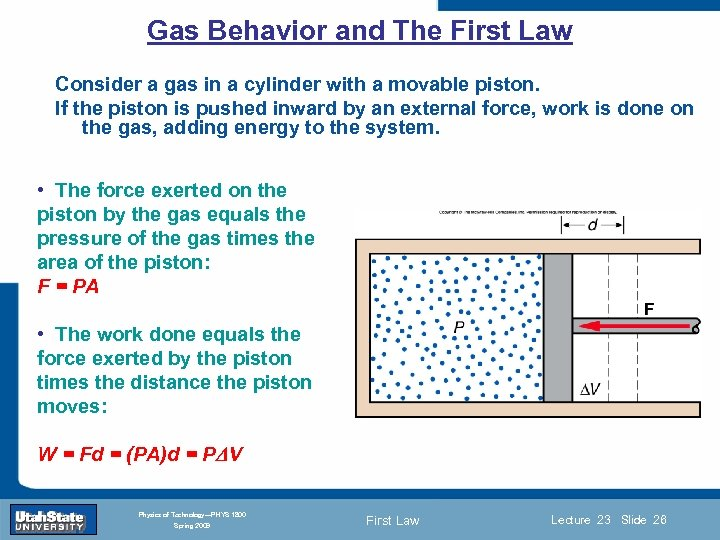Gas Behavior and The First Law Consider a gas in a cylinder with a movable piston. If the piston is pushed inward by an external force, work is done on the gas, adding energy to the system. • The force exerted on the piston by the gas equals the pressure of the gas times the area of the piston: F = PA • The work done equals the force exerted by the piston times the distance the piston Introduction Section 0 Lecture 1 moves: Slide 26 W = Fd = (PA)d = P V INTRODUCTION TO Modern Physics PHYX 2710 Fall 2004 Physics of Technology—PHYS 1800 Spring 2009 First Law Lecture 23 Slide 26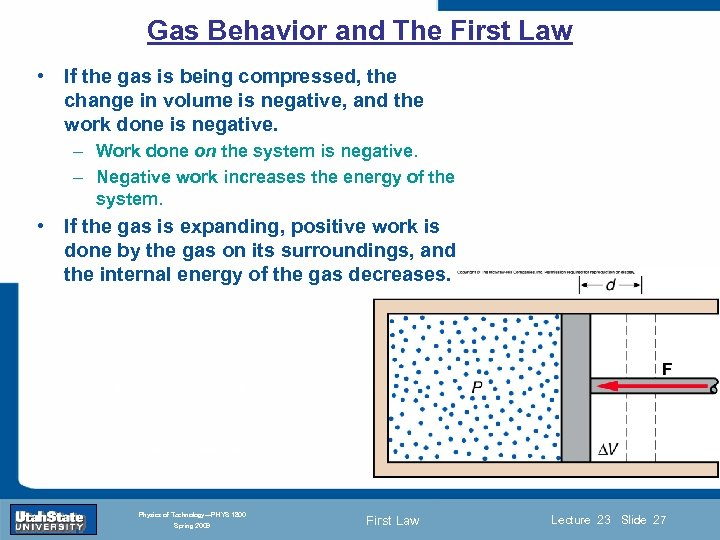Gas Behavior and The First Law • If the gas is being compressed, the change in volume is negative, and the work done is negative. – Work done on the system is negative. – Negative work increases the energy of the system. • If the gas is expanding, positive work is done by the gas on its surroundings, and the internal energy of the gas decreases. Introduction Section 0 Lecture 1 Slide 27 INTRODUCTION TO Modern Physics PHYX 2710 Fall 2004 Physics of Technology—PHYS 1800 Spring 2009 First Law Lecture 23 Slide 27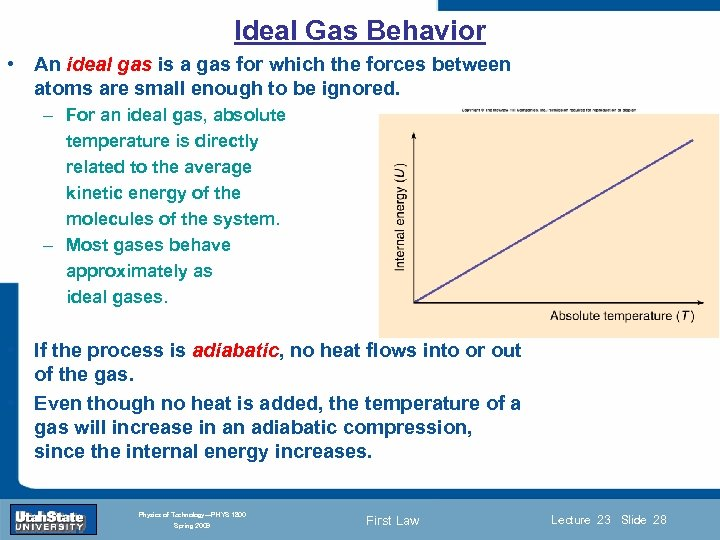Ideal Gas Behavior • An ideal gas is a gas for which the forces between atoms are small enough to be ignored. – For an ideal gas, absolute temperature is directly related to the average kinetic energy of the molecules of the system. – Most gases behave approximately as ideal gases. • If the process is adiabatic, no heat flows into or out of the gas. Introduction Section 0 Lecture 1 Slide 28 • Even though no heat is added, the temperature of a gas will increase in an adiabatic compression, since the internal energy increases. INTRODUCTION TO Modern Physics PHYX 2710 Fall 2004 Physics of Technology—PHYS 1800 Spring 2009 First Law Lecture 23 Slide 28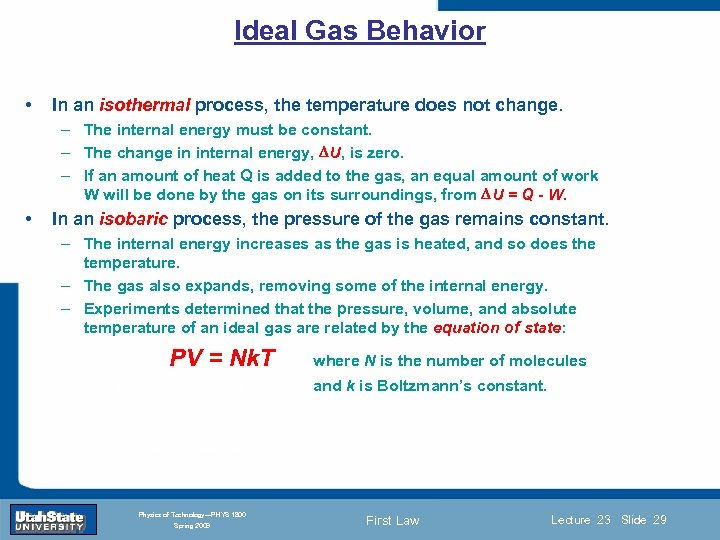Ideal Gas Behavior • In an isothermal process, the temperature does not change. – The internal energy must be constant. – The change in internal energy, U, is zero. – If an amount of heat Q is added to the gas, an equal amount of work W will be done by the gas on its surroundings, from U = Q - W. • In an isobaric process, the pressure of the gas remains constant. – The internal energy increases as the gas is heated, and so does the temperature. – The gas also expands, removing some of the internal energy. – Experiments determined that the pressure, volume, and absolute temperature of an ideal gas are related by the equation of state: PV = Nk. T Introduction Section 0 where N is the number of molecules Lecture 1 and k is Boltzmann’s Slide 29 constant. INTRODUCTION TO Modern Physics PHYX 2710 Fall 2004 Physics of Technology—PHYS 1800 Spring 2009 First Law Lecture 23 Slide 29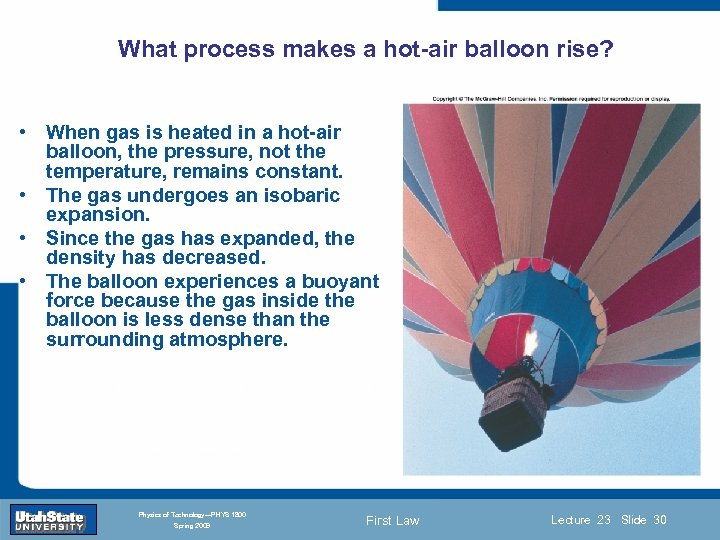What process makes a hot-air balloon rise? • When gas is heated in a hot-air balloon, the pressure, not the temperature, remains constant. • The gas undergoes an isobaric expansion. • Since the gas has expanded, the density has decreased. • The balloon experiences a buoyant force because the gas inside the balloon is less dense than the surrounding atmosphere. Introduction Section 0 Lecture 1 Slide 30 INTRODUCTION TO Modern Physics PHYX 2710 Fall 2004 Physics of Technology—PHYS 1800 Spring 2009 First Law Lecture 23 Slide 30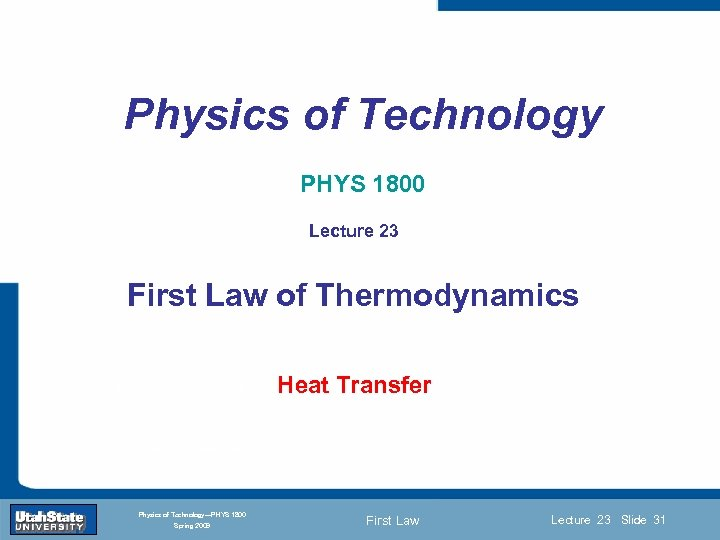Physics of Technology PHYS 1800 Lecture 23 First Law of Thermodynamics Introduction Section 0 Heat. Slide 31 Transfer Lecture 1 INTRODUCTION TO Modern Physics PHYX 2710 Fall 2004 Physics of Technology—PHYS 1800 Spring 2009 First Law Lecture 23 Slide 31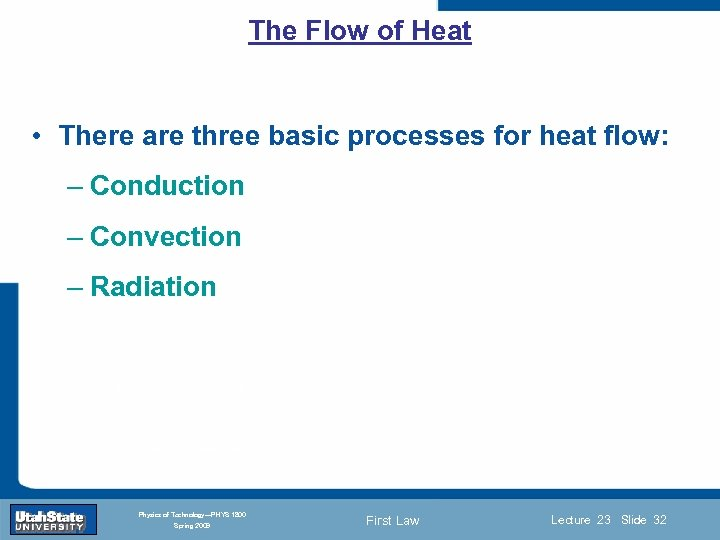The Flow of Heat • There are three basic processes for heat flow: – Conduction – Convection – Radiation Introduction Section 0 Lecture 1 Slide 32 INTRODUCTION TO Modern Physics PHYX 2710 Fall 2004 Physics of Technology—PHYS 1800 Spring 2009 First Law Lecture 23 Slide 32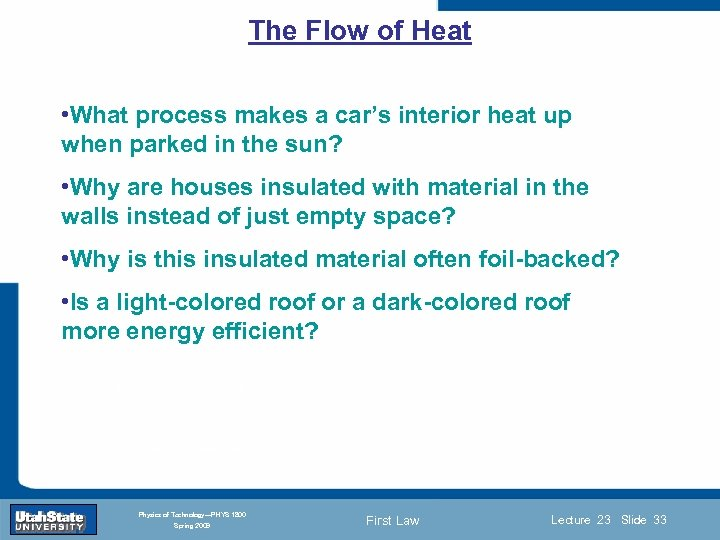The Flow of Heat • What process makes a car’s interior heat up when parked in the sun? • Why are houses insulated with material in the walls instead of just empty space? • Why is this insulated material often foil-backed? • Is a light-colored roof or a dark-colored roof more energy efficient? Introduction Section 0 Lecture 1 Slide 33 INTRODUCTION TO Modern Physics PHYX 2710 Fall 2004 Physics of Technology—PHYS 1800 Spring 2009 First Law Lecture 23 Slide 33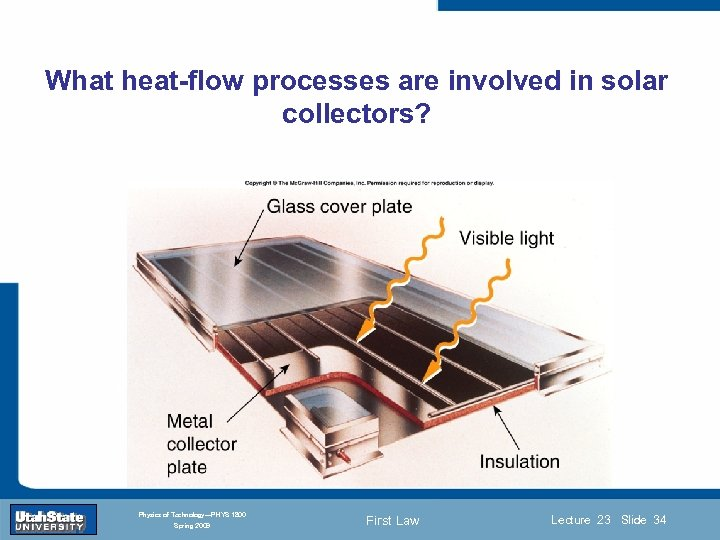What heat-flow processes are involved in solar collectors? Introduction Section 0 Lecture 1 Slide 34 INTRODUCTION TO Modern Physics PHYX 2710 Fall 2004 Physics of Technology—PHYS 1800 Spring 2009 First Law Lecture 23 Slide 34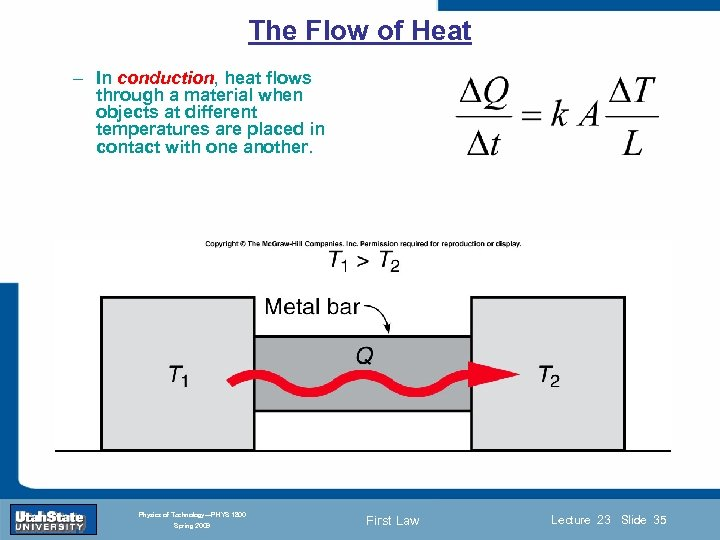The Flow of Heat – In conduction, heat flows through a material when objects at different temperatures are placed in contact with one another. Introduction Section 0 Lecture 1 Slide 35 INTRODUCTION TO Modern Physics PHYX 2710 Fall 2004 Physics of Technology—PHYS 1800 Spring 2009 First Law Lecture 23 Slide 35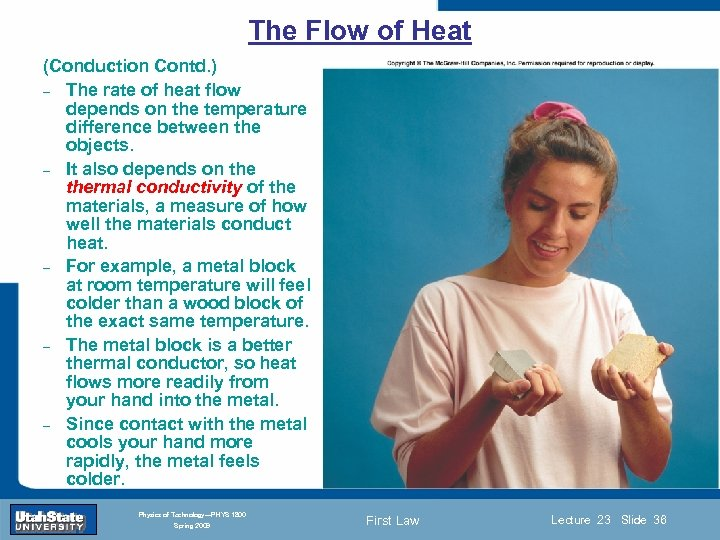The Flow of Heat (Conduction Contd. ) – The rate of heat flow depends on the temperature difference between the objects. – It also depends on thermal conductivity of the materials, a measure of how well the materials conduct heat. – For example, a metal block at room temperature will feel colder than a wood block of the exact same temperature. – The metal block is a better thermal conductor, so heat flows more readily from Introduction Section 0 Lecture 1 your hand into the metal. – Since contact with the metal cools your hand more rapidly, the metal feels colder. Slide 36 INTRODUCTION TO Modern Physics PHYX 2710 Fall 2004 Physics of Technology—PHYS 1800 Spring 2009 First Law Lecture 23 Slide 36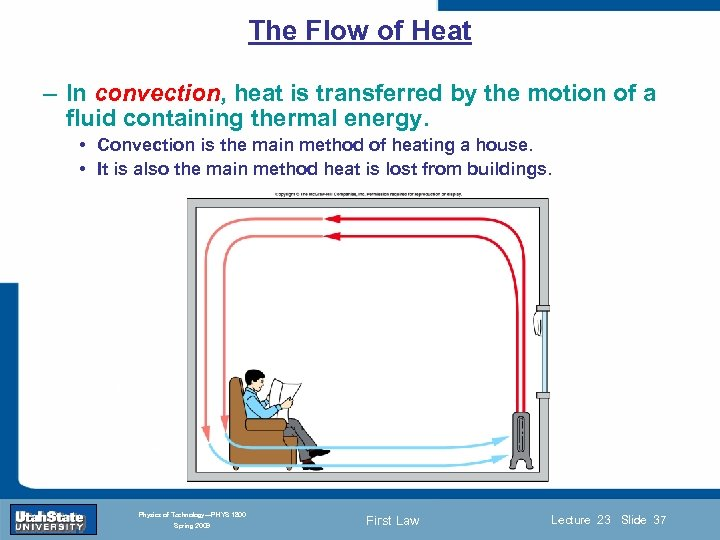The Flow of Heat – In convection, heat is transferred by the motion of a fluid containing thermal energy. • Convection is the main method of heating a house. • It is also the main method heat is lost from buildings. Introduction Section 0 Lecture 1 Slide 37 INTRODUCTION TO Modern Physics PHYX 2710 Fall 2004 Physics of Technology—PHYS 1800 Spring 2009 First Law Lecture 23 Slide 37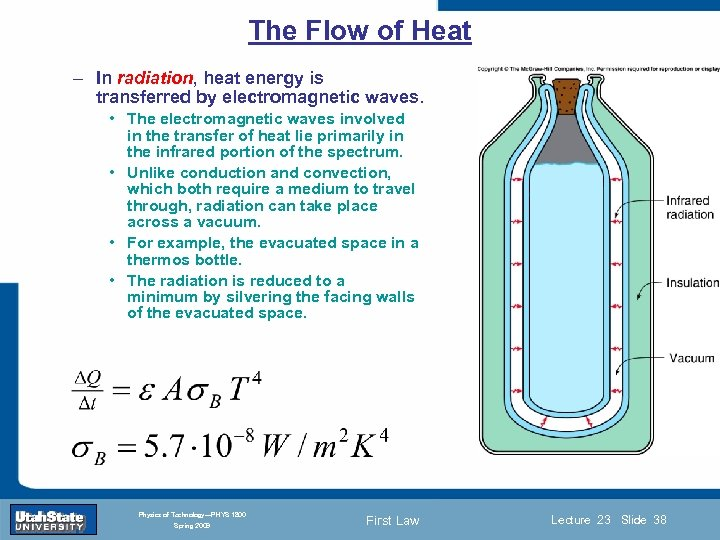The Flow of Heat – In radiation, heat energy is transferred by electromagnetic waves. • The electromagnetic waves involved in the transfer of heat lie primarily in the infrared portion of the spectrum. • Unlike conduction and convection, which both require a medium to travel through, radiation can take place across a vacuum. • For example, the evacuated space in a thermos bottle. • The radiation is reduced to a minimum by silvering the facing walls of the evacuated space. Introduction Section 0 Lecture 1 Slide 38 INTRODUCTION TO Modern Physics PHYX 2710 Fall 2004 Physics of Technology—PHYS 1800 Spring 2009 First Law Lecture 23 Slide 38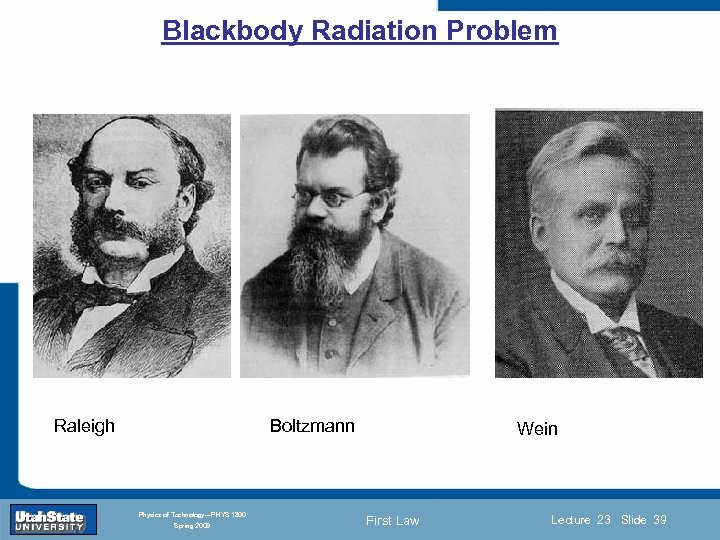Blackbody Radiation Problem Introduction Section 0 Raleigh Lecture 1 Slide 39 Boltzmann Wein INTRODUCTION TO Modern Physics PHYX 2710 Fall 2004 Physics of Technology—PHYS 1800 Spring 2009 First Law Lecture 23 Slide 39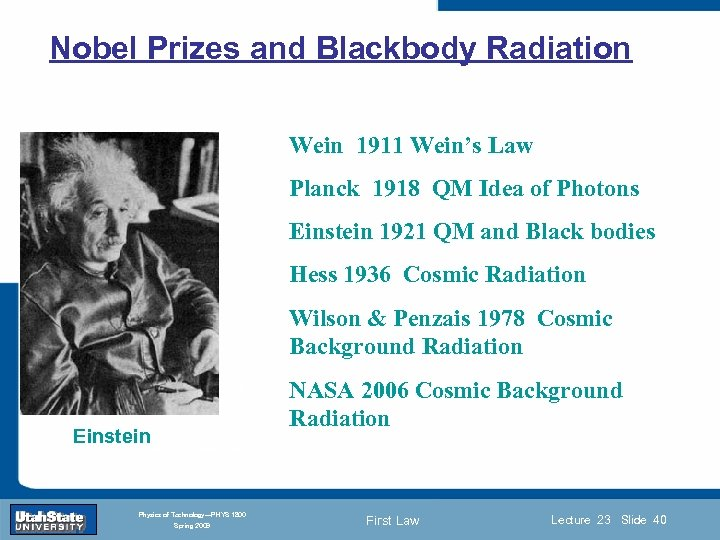Nobel Prizes and Blackbody Radiation Wein 1911 Wein’s Law Planck 1918 QM Idea of Photons Einstein 1921 QM and Black bodies Hess 1936 Cosmic Radiation Wilson & Penzais 1978 Cosmic Background Radiation Introduction Section 0 Einstein Slide 40 NASA 2006 Cosmic Background Radiation Lecture 1 INTRODUCTION TO Modern Physics PHYX 2710 Fall 2004 Physics of Technology—PHYS 1800 Spring 2009 First Law Lecture 23 Slide 40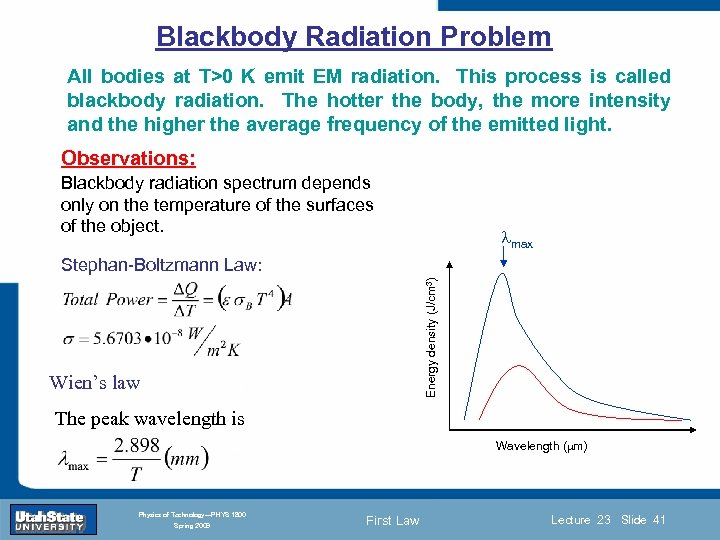Blackbody Radiation Problem All bodies at T>0 K emit EM radiation. This process is called blackbody radiation. The hotter the body, the more intensity and the higher the average frequency of the emitted light. Observations: Blackbody radiation spectrum depends only on the temperature of the surfaces of the object. lmax Wien’s law Introduction Section 0 Lecture 1 Slide 41 Energy density (J/cm 3) Stephan-Boltzmann Law: The peak wavelength is Wavelength (mm) INTRODUCTION TO Modern Physics PHYX 2710 Fall 2004 Physics of Technology—PHYS 1800 Spring 2009 First Law Lecture 23 Slide 41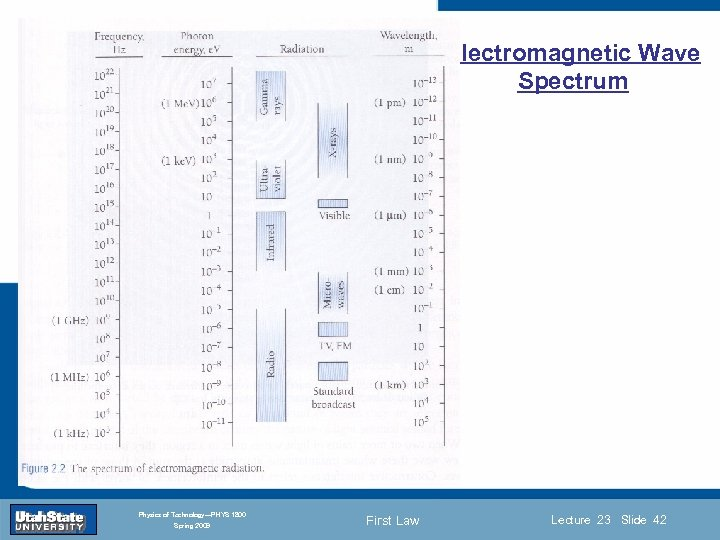Electromagnetic Wave Spectrum Introduction Section 0 Lecture 1 Slide 42 INTRODUCTION TO Modern Physics PHYX 2710 Fall 2004 Physics of Technology—PHYS 1800 Spring 2009 First Law Lecture 23 Slide 42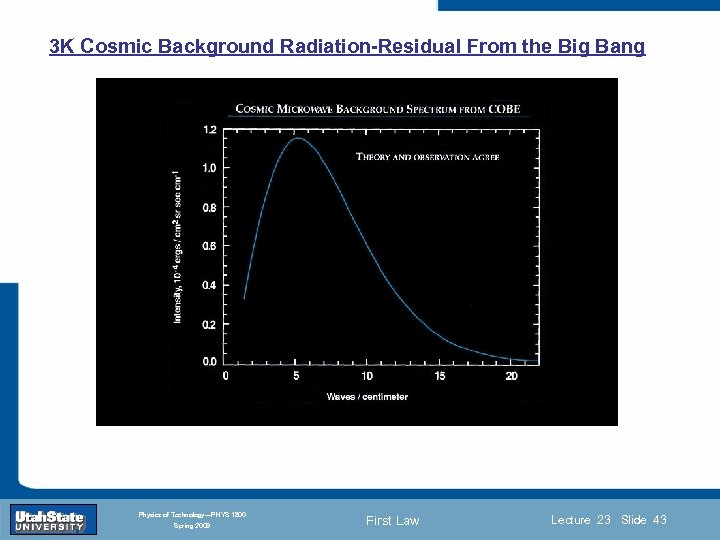3 K Cosmic Background Radiation-Residual From the Big Bang Introduction Section 0 Lecture 1 Slide 43 INTRODUCTION TO Modern Physics PHYX 2710 Fall 2004 Physics of Technology—PHYS 1800 Spring 2009 First Law Lecture 23 Slide 43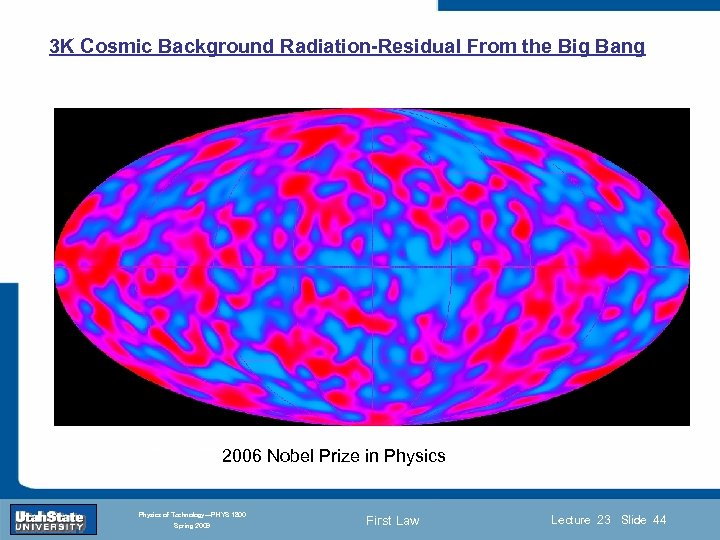3 K Cosmic Background Radiation-Residual From the Big Bang Introduction Section 0 Lecture 1 Slide 44 INTRODUCTION TO Modern Physics PHYX 2710 Fall 2004 2006 Nobel Prize in Physics of Technology—PHYS 1800 Spring 2009 First Law Lecture 23 Slide 44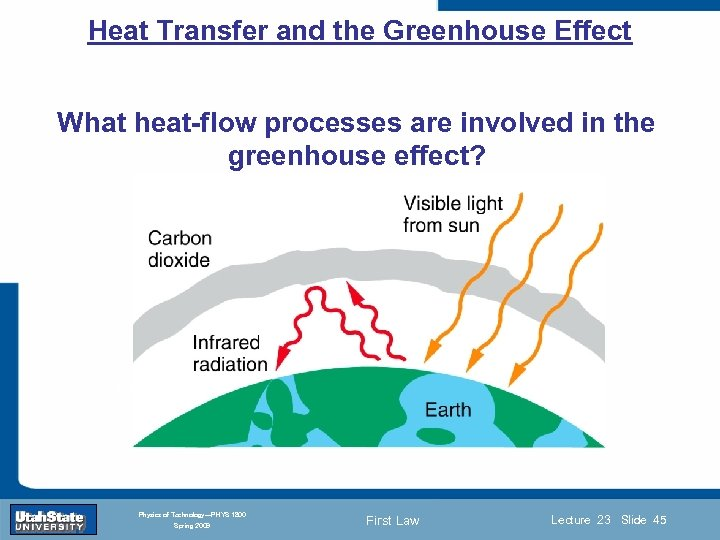Heat Transfer and the Greenhouse Effect What heat-flow processes are involved in the greenhouse effect? Introduction Section 0 Lecture 1 Slide 45 INTRODUCTION TO Modern Physics PHYX 2710 Fall 2004 Physics of Technology—PHYS 1800 Spring 2009 First Law Lecture 23 Slide 45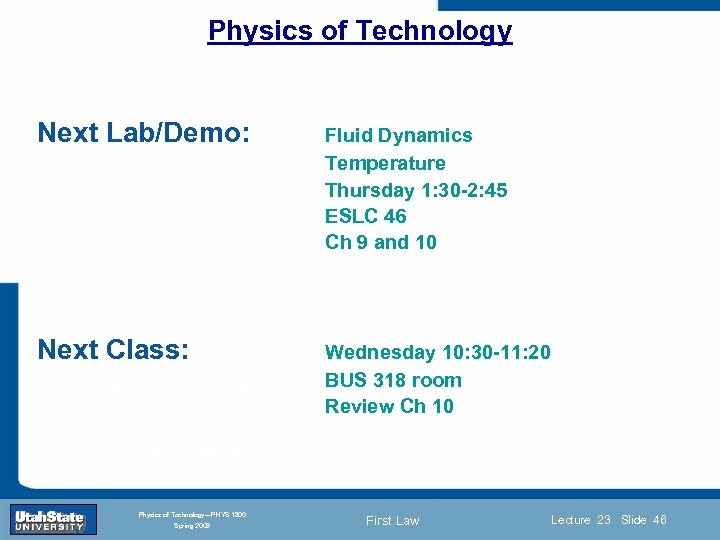Physics of Technology Next Lab/Demo: Fluid Dynamics Temperature Thursday 1: 30 -2: 45 ESLC 46 Ch 9 and 10 Next Class: Wednesday 10: 30 -11: 20 BUS 318 room Slide 46 Review Ch 10 Introduction Section 0 Lecture 1 INTRODUCTION TO Modern Physics PHYX 2710 Fall 2004 Physics of Technology—PHYS 1800 Spring 2009 First Law Lecture 23 Slide 46